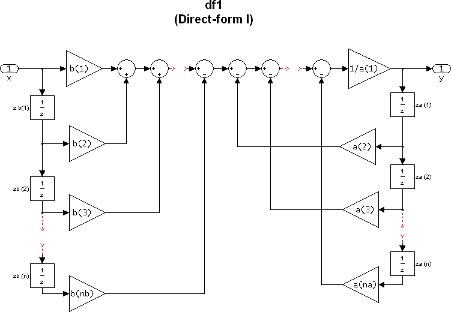# dfilt.df1

Discrete-time, direct-form I filter

## Syntax

```Hd = dfilt.df1(b,a) Hd = dfilt.df1 ```

## Description

`Hd = dfilt.df1(b,a)` returns a discrete-time, direct-form I filter, `Hd`, with numerator coefficients `b` and denominator coefficients `a`. The filter states for this object are stored in a `filtstates` object.

`Hd = dfilt.df1` returns a default, discrete-time, direct-form I filter, `Hd`, with `b`=1 and `a`=1. This filter passes the input through to the output unchanged.

Note

The leading coefficient of the denominator `a(1)` cannot be 0.

Image of direct form one filter diagramTo display the filter states, use this code to access the `filtstates` object.

```Hs = Hd.states % Where Hd is the dfilt.df1 object and double (Hs) % Hs is the filtstates object ```

The vector is

`$\left[\begin{array}{c}zb\left(1\right)\\ zb\left(2\right)\\ \cdots \\ zb\left(n\right)\\ za\left(1\right)\\ za\left(2\right)\\ \cdots \\ za\left(n\right)\end{array}\right]$`

## Examples

Create a direct-form I discrete-time filter with coefficients from a fourth-order lowpass Butterworth design

```[b,a] = butter(4,.5); Hd = dfilt.df1(b,a) ```